# Consider the following differential equation. (1 + 5x2) y′′ − 8xy′ − 6y  =  0 (a)...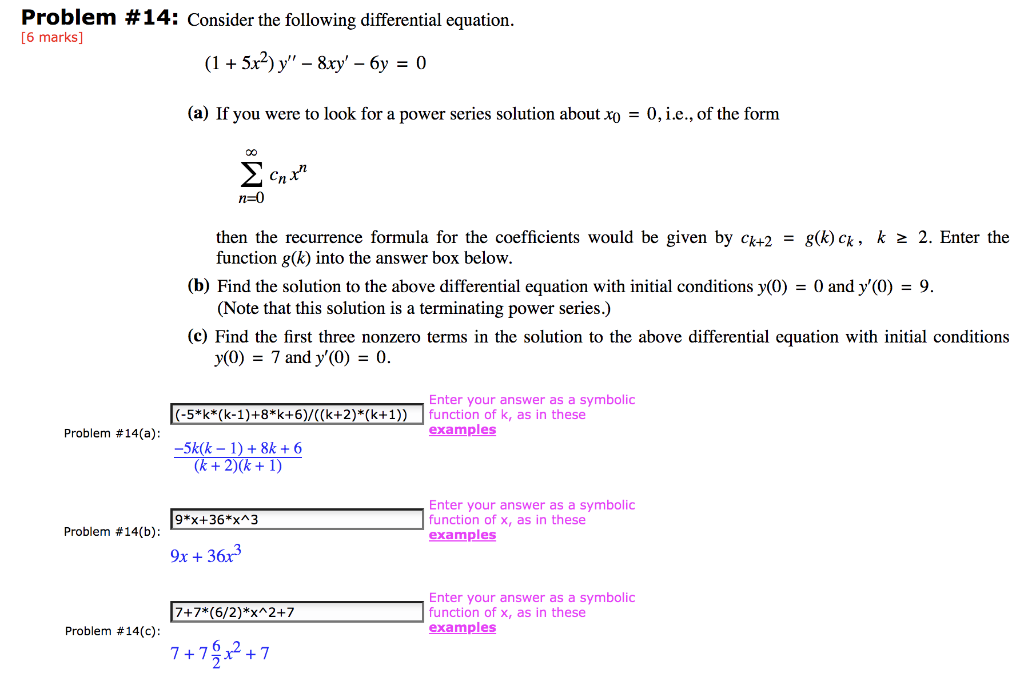Consider the following differential equation. (1 + 5x2) y′′ − 8xy′ − 6y  =  0
(a) If you were to look for a power series solution about x0  =  0, i.e., of the form
 ∞ Σ n=0
cnxn

then the recurrence formula for the coefficients would be given by ck+2  =  g(k) ck , k  ≥  2. Enter the function g(k) into the answer box below.
 (b) Find the solution to the above differential equation with initial conditions y(0)  =  0 and y′(0)  =  9. (Note that this solution is a terminating power series.)
 (c) Find the first three nonzero terms in the solution to the above differential equation with initial conditions y(0)  =  7 and y′(0)  =  0.
Problem #14: Consider the following differential equation. [6 marks] (1 + 5x2)y" – 8xy' – 6y = 0 (a) If you were to look for a power series solution about xo = 0, i.e., of the form Σc, Cnxh n=0 then the recurrence formula for the coefficients would be given by Ck+2 = g(k) Ck, k > 2. Enter the function gk) into the answer box below. (b) Find the solution to the above differential equation with initial conditions y(0) = 0 and y'(0) = 9. (Note that this solution is a terminating power series.) (c) Find the first three nonzero terms in the solution to the above differential equation with initial conditions y(O) = 7 and y'(0) = 0. (-5*k*(k-1)+8*+6)/((k+2)*(k+1)) Enter your answer as a symbolic function of k, as in these examples Problem #14(a): -5k(k – 1) + 8 + 6 (k + 2)(k + 1) 9*x+36*x^3 Enter your answer as a symbolic function of x, as in these examples Problem #14(b): 9x + 3683 7+7*(6/2)*x^2+7 Enter your answer as a symbolic function of X, as in these examples Problem #14(c): 7+7Qx2 +7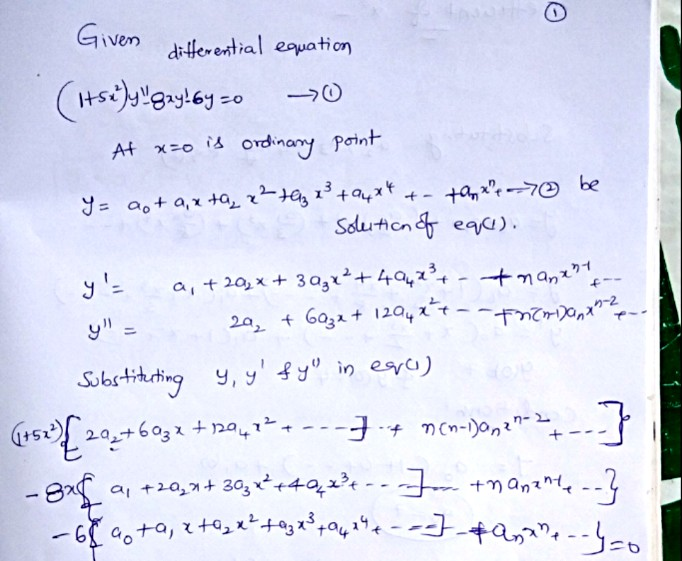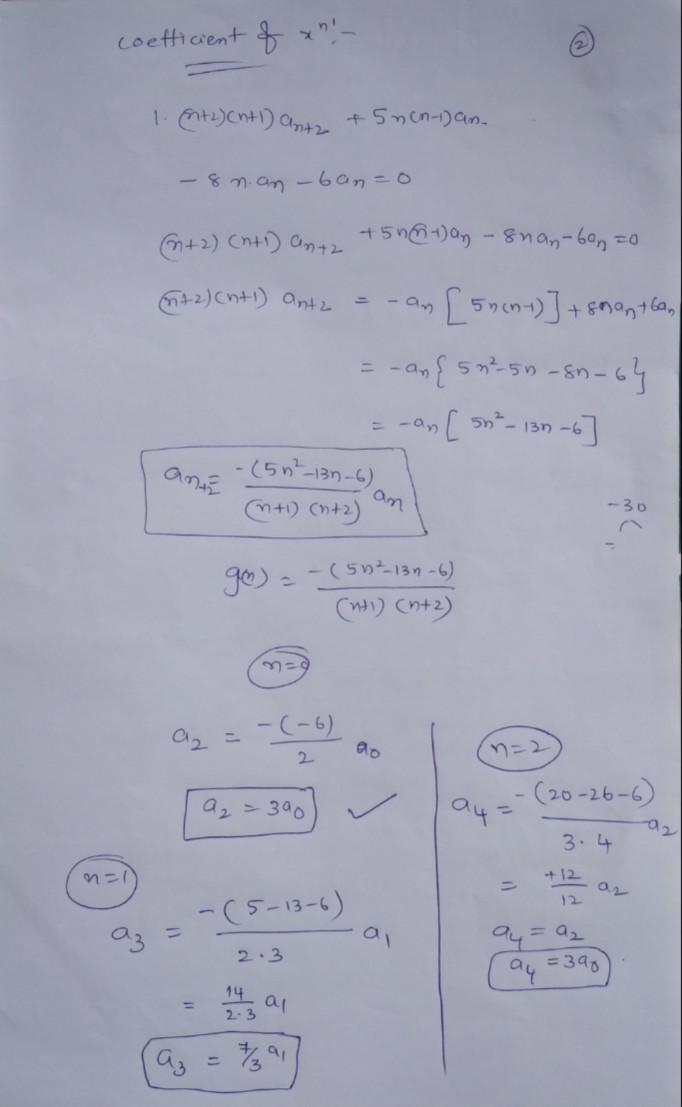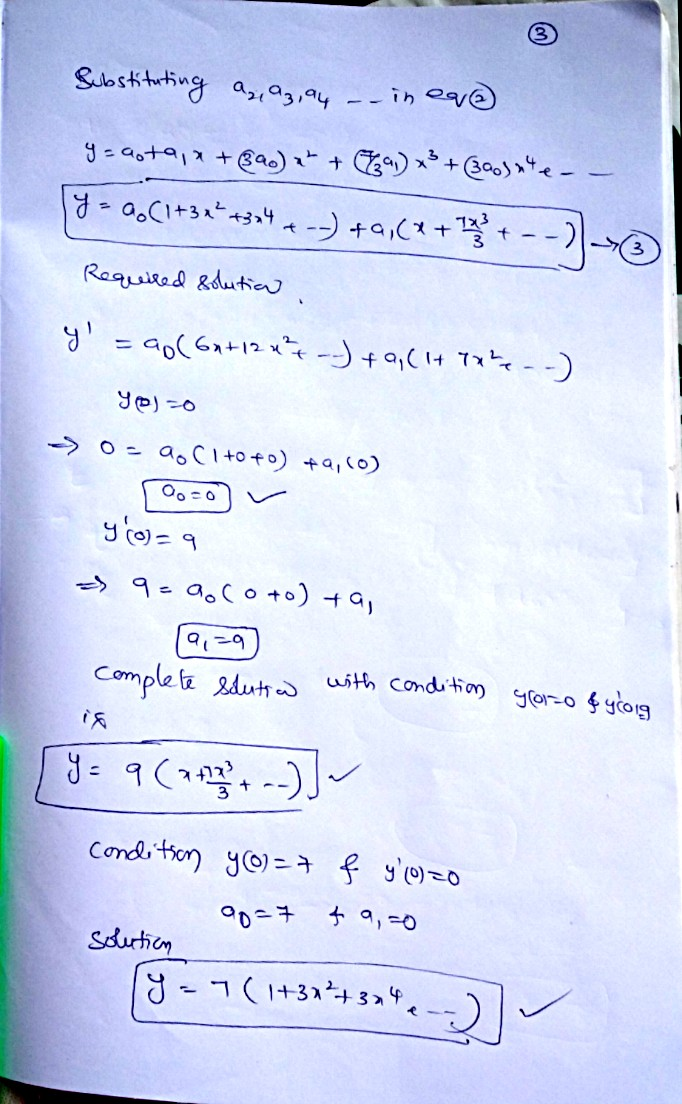##### Add Answer of: Consider the following differential equation. (1 + 5x2) y′′ − 8xy′ − 6y  =  0 (a)...
Similar Homework Help Questions
• ### Consider the following differential equation. (1 + 5x2)y" – 8xY' – 6y = 0 (a) If...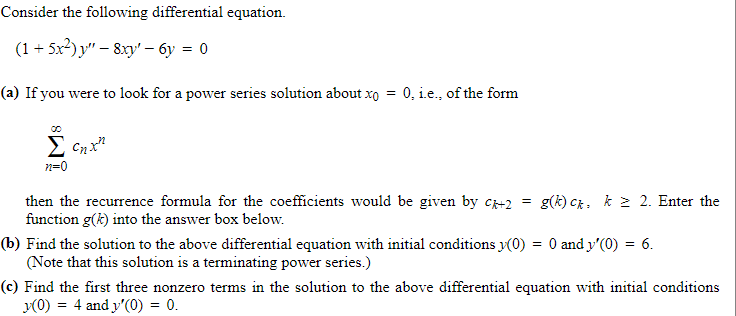Consider the following differential equation. (1 + 5x2)y" – 8xY' – 6y = 0 (a) If you were to look for a power series solution about xo = 0, i.e., of the form Σ τη x2 n=0 then the recurrence formula for the coefficients would be given by C +2 g(k) Cx. k > 2. Enter the function g(k) into the answer box below. (b) Find the solution to the above differential equation with initial conditions (0) = 0 and...

• ### Consider the following differential equation. (1 + 6x2)y" – 4xy' – 24y = 0 (a) If...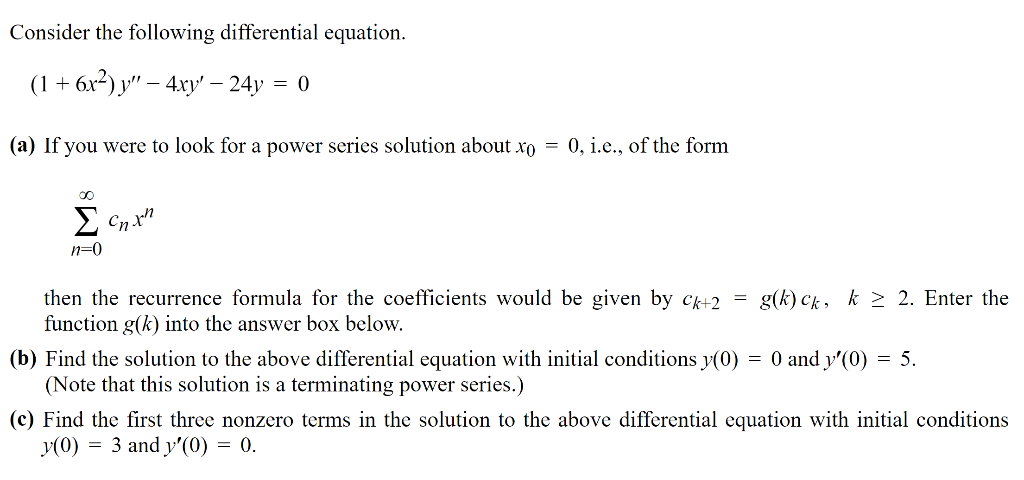Consider the following differential equation. (1 + 6x2)y" – 4xy' – 24y = 0 (a) If you were to look for a power series solution about xo = 0, i.e., of the form onth n=0 then the recurrence formula for the coefficients would be given by ck+2 = g(k) Ck, k > 2. Enter the function g(k) into the answer box below. (b) Find the solution to the above differential equation with initial conditions y(0) = 0 and y'(0) =...

• ### cnrn Consider the following differential equation. (1 + 3x?) y" – 2xy' – 12y = 0...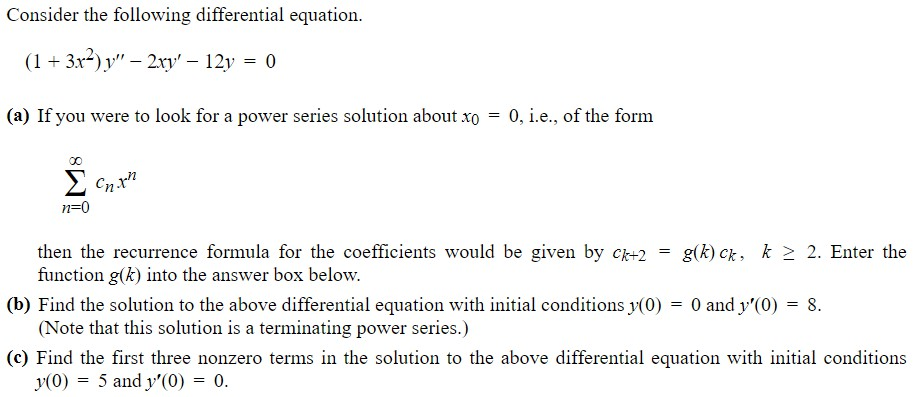cnrn Consider the following differential equation. (1 + 3x?) y" – 2xy' – 12y = 0 (a) If you were to look for a power series solution about xo = 0, i.e., of the form Σ n=0 00 then the recurrence formula for the coefficients would be given by Ck+2 g(k) Ck, k > 2. Enter the function g(k) into the answer box below. (b) Find the solution to the above differential equation with initial conditions y(0) = 0 and...

• ### Consider the following initial value problem, (1 - z2)y"+zy' - 12y-0, (0)3, y' (0)-0. Note: For each part b...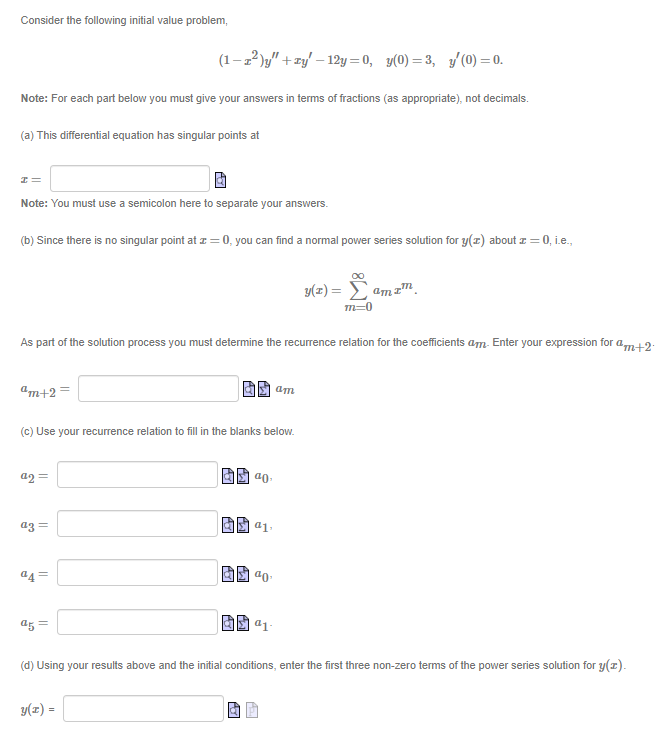Consider the following initial value problem, (1 - z2)y"+zy' - 12y-0, (0)3, y' (0)-0. Note: For each part below you must give your answers in terms of fractions (as appropriate), not decimals (a) This differential equation has singular points at Note: You must use a semicolon here to separate your answers. (b) Since there is no singular point at z 0, you can find a normal power series solution for y(x about z0,i.e. m-0 As part of the solution process...

• ### Consider the following differential equation to be solved using a power series. y" - y' =...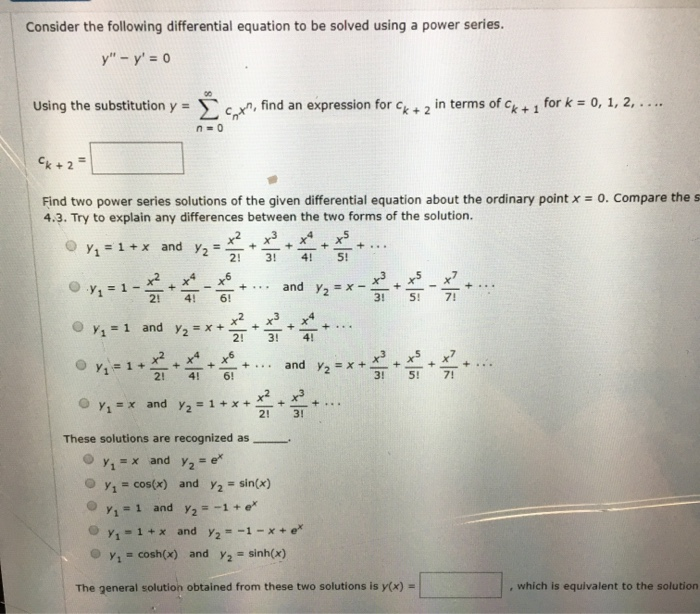Consider the following differential equation to be solved using a power series. y" - y' = 0 Using the substitution y = į coxn, find an expression for Ck + 2 in terms of Ck + 1 for k = 0, 1, 2, .... k+2= + + + Find two power series solutions of the given differential equation about the ordinary point x = 0. Compare the s 4.3. Try to explain any differences between the two forms of the...

• ### 7. Consider the differential equation (a) Show that z 0 is a regular singular point of the above differential equation (b) Let y(x) be a solution of the differential equation, where r R and the s...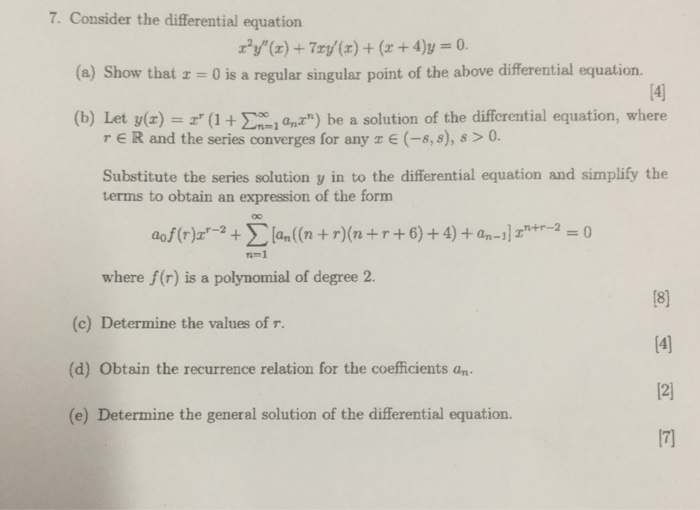7. Consider the differential equation (a) Show that z 0 is a regular singular point of the above differential equation (b) Let y(x) be a solution of the differential equation, where r R and the series converges for any E (-8,s), s > 0 Substitute the series solution y in to the differential equation and simplify the terms to obtain an expression of the form 1-1 where f(r) is a polynomial of degree 2. (c) Determine the values of r....

• ### (1 point) In this problem you will solve the differential equation (+7)y"+11xy' - y=0. x" for...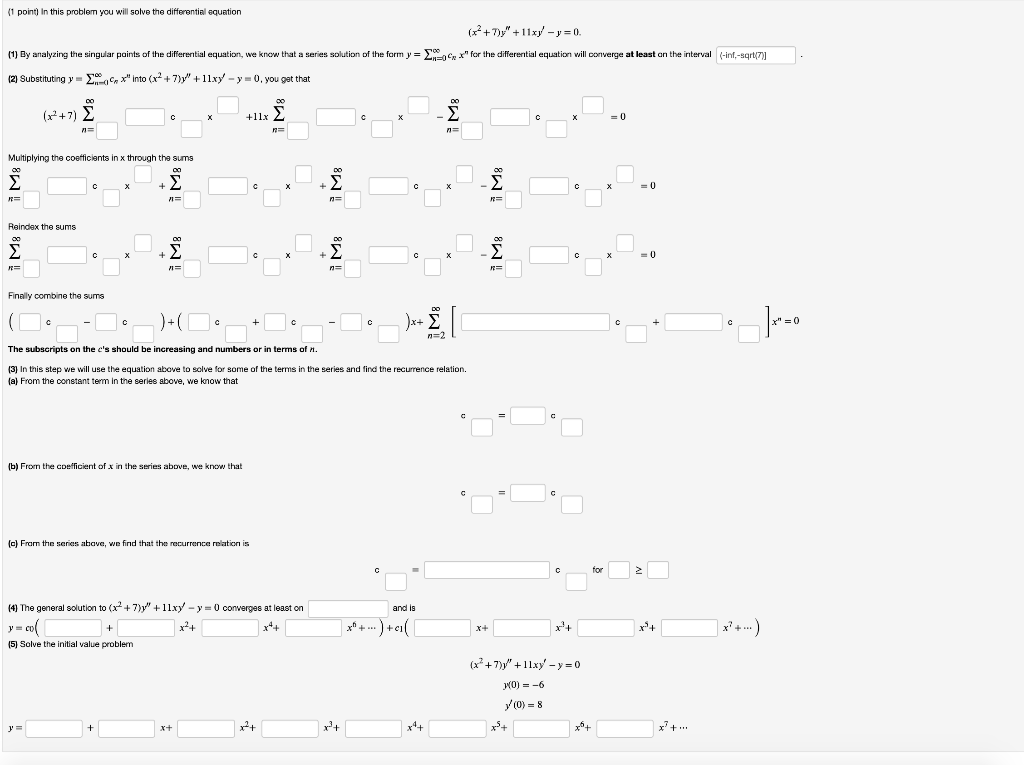(1 point) In this problem you will solve the differential equation (+7)y"+11xy' - y=0. x" for the differential equation will converge at least on the interval (-inf.-sqrt(7)] (1) Ey analyzing the singular paints of the differential equation, we know that a series solution of the form y = . (2) Substituting y = . *" into (x2+7y" + 11xy - y = 0, you get that Multiplying the coefficients in x through the sums E Reindex the sums Finally combine...

• ### (1) Sok power series solution of the forma y(z)-Σ-oanz" to the differential equation: (a) (3 pts) Find recurrence relations for the coefficents, an (b) (4 pts) Use the recurrence relation to g...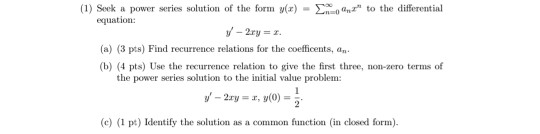(1) Sok power series solution of the forma y(z)-Σ-oanz" to the differential equation: (a) (3 pts) Find recurrence relations for the coefficents, an (b) (4 pts) Use the recurrence relation to give the first three, n-zero terms of the power series solution to the initial value problem: y'-2xy = z, y(0) = 2 (c) (1 pt) Identify the solution as a common function (in closed form). (1) Sok power series solution of the forma y(z)-Σ-oanz" to the differential equation: (a)...

• ### 3. Consider the following differential equation 0o and a series solution to the differential equation of the form a&#34...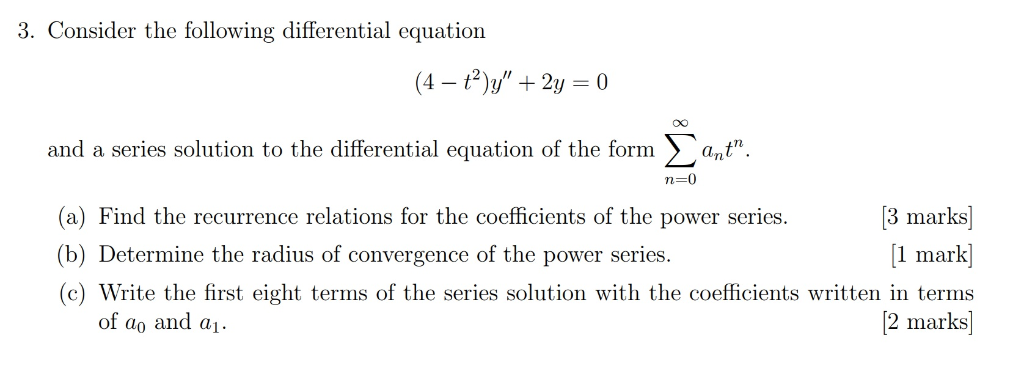3. Consider the following differential equation 0o and a series solution to the differential equation of the form a" n-0 (a) Find the recurrence relations for the coefficients of the power series. 3 marks] (b) Determine the radius of convergence of the power series. l mar (c) Write the first eight terms of the series solution with the coefficients written in terms of ao and ai 2 marks] 3. Consider the following differential equation 0o and a series solution to...

• ### differential equations Consider the following differential equation to be solved using a power series. y" +...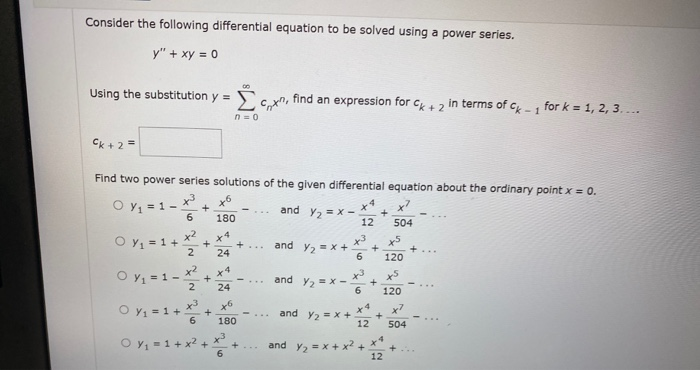differential equations Consider the following differential equation to be solved using a power series. y" + xy = 0 On Using the substitution y = cryn, find an expression for Ck + 2 in terms of Ck - 1 for k = 1, 2, 3... n = 0 Ck +2= + 6 Find two power series solutions of the given differential equation about the ordinary point x = 0. x3 O Y1 = 1 - xo and y2 = x...

Free Homework App